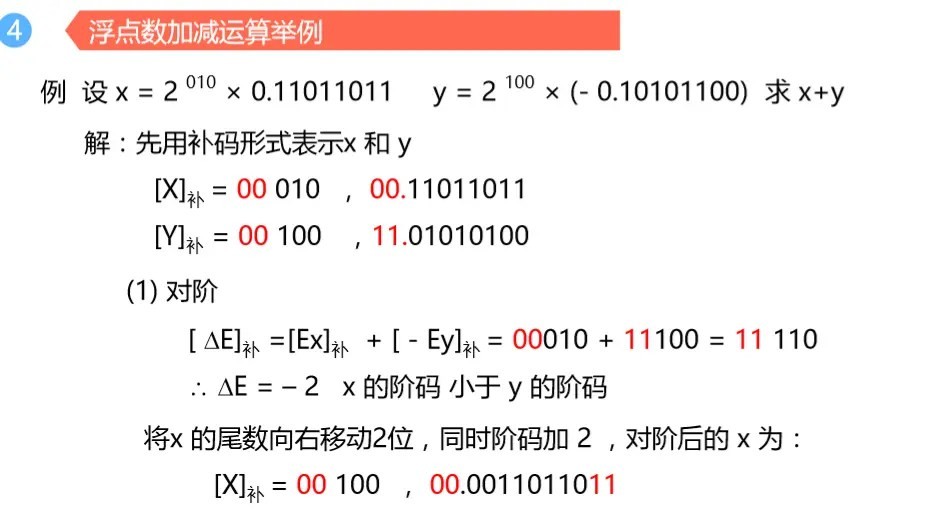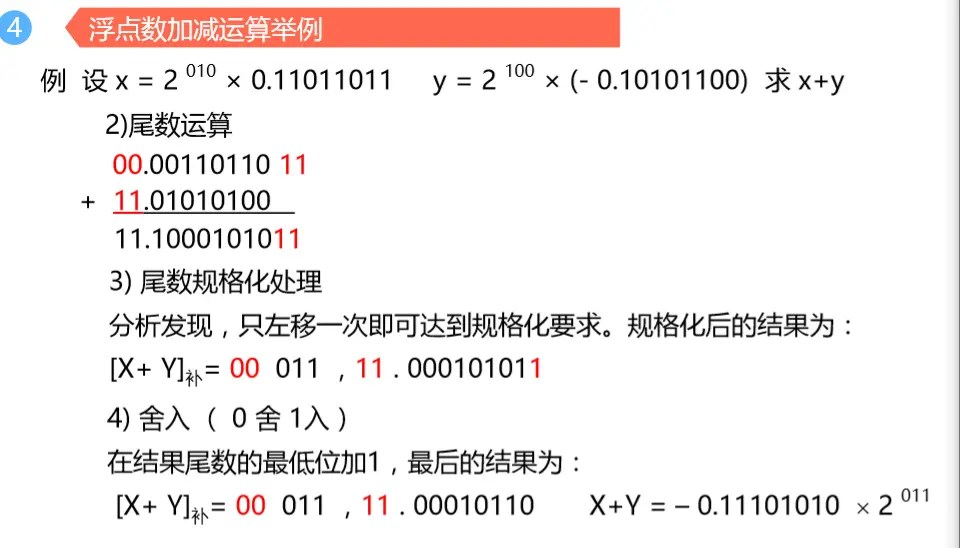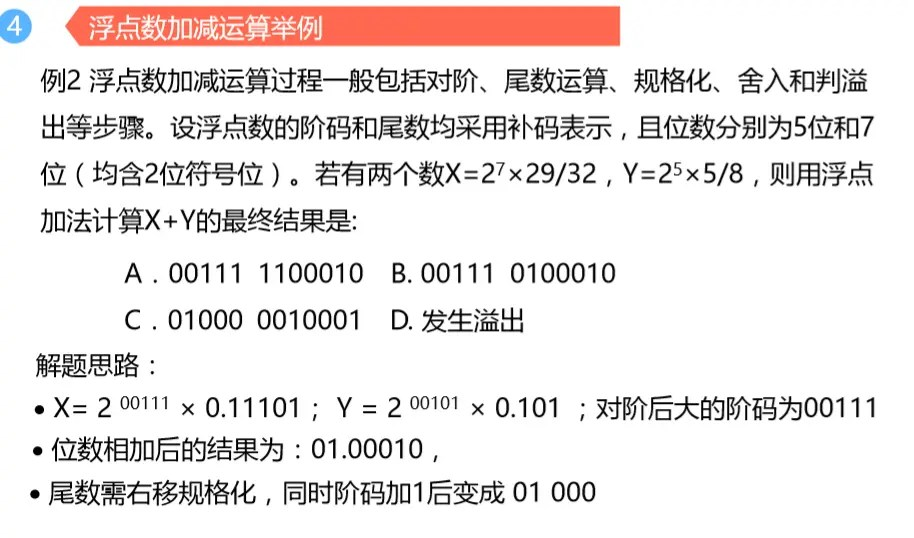# Principles of Computer 3.7 Addition and subtraction of floating-point numbers

Posted Jun 16, 20202 min read

1. The concept of normalized floating point numbers

Since floating-point numbers are data representation methods that separately represent the range and precision of data, unless the floating-point numbers are explicitly specified, the same floating-point number is not unique.

Normalized floating-point numbers refer to converting a floating-point number into a specified format.

Taking the general format of floating-point numbers as an example, the form of the mantissa of normalized floating-point numbers is:1. Normalization method of floating point numbers

When the result of the mantissa is 00.0····or 11.1···, the left normalization is required to move the mantissa to the left, and each time it moves, the order code is decreased by 1 until the form of the mantissa is 00.1···or 11.0···

When the result of the mantissa is 01.··· or 10.···, indicating that the result of the mantissa summation is >1, only one right shift normalization needs to be performed at this time, the order code is increased by 1, and the form of the mantissa is 00.1··· Or 11.0...

1. Addition and subtraction methods and steps of floating point numbers1) Pair order

Find the difference

Right shift the mantissa of the floating point number with a small order code and increase its order code synchronously until the two order codes are equal.

Mantissa addition/subtraction(using the mantissa after the order)

3) Normalization of results

4) Rounding

When shifting to the right, some low-order values may be lost. To improve accuracy, a rounding method can be adopted

0 round 1 round:if the right shift is 1 then add 1 to the lowest bit

Permanently set 1:As long as the digital bit 1 is removed, the last bit is permanently set to 1.

5) Overflow handling

Floating-point overflow flag:order code overflow

Order code overflow:the sign bit of order code is 01

Order code underflow:the sign bit of order code is 10